## Thursday, May 18, 2006

### Curse of Dimensionality and intuition

There are some counter-intuitive things happening as dimension increases.

For instance consider unit sphere (radius 1)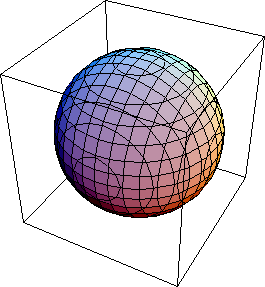If you go from 2 dimensions to 3 dimensions, the volume increases (Pi to 4/3 pi). Similar increase happens when you go to 3,4,5 dimensions. But then the volume starts to decrease. Eventually it decreases all the way to 0.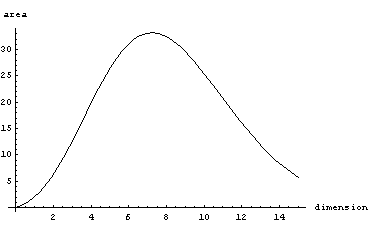Now consider a cube of width 1. As dimension increases, the volume stays the same. But the length of the main diagonal grows as sqrt(dimension). So the corners become situated further and further away from the center, and eventually almost all the mass is concentrated in the corners (meaning outside of the inscribed sphere)

Here's a plot of the fraction of the mass concentrated in the corners as a function of dimension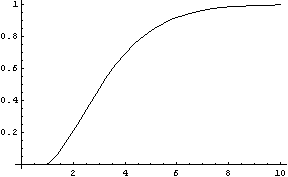For a 7 dimensional cube, about 96% of the mass is concentrated in one of it's 128 "corners", so if we had 7 dimensional vision, we might see that cube like this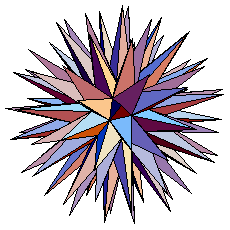Finally consider what happens with a d-dimensional standard normal (unit covariance matrix, centered at 0). If we plot probability mass contained around a thin shell of radius r as a function r we get the following graphs (d=1,d=4,d=16)

The mean of this distribution is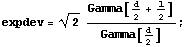which can be approximated as sqrt(d)

Furthermore you can derive that variance of this distribution converges to 1/2 (see notebook). Applying Chebyshev's inequality, we get that for any dimension, at least 0.75 of the mass is contained in the shell of thickness 1 centered at about sqrt(d). In numerical integration this seems to converge to a slightly higher number, for instance for 10^6 dimensions, it's about 0.84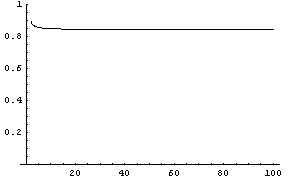Here's the Mathematica notebook with some derivations (web version)

## Tuesday, May 09, 2006

### Positive-definite/negative-definite

Concepts of positive definite matrices and positive definite operators often come up in Machine Learning, here are some movies I made to visualize linear transformations corresponding to some common types of matrices:

Symmetric and positive definite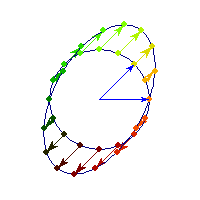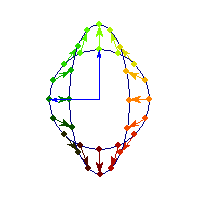Symmetric positive definite matrices can be thought of matrices that stretch or shrink the circle along the eigenvectors (marked in blue).

The angle between x and Ax is always less than Pi/2, so x'Ax>0 forall x.

Symmetric and negative definite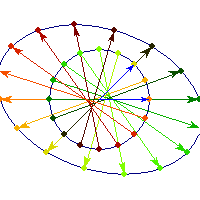You can see in addition to stretching, every vector is flipped across the origin. So the angle is always between Pi/2 and Pi, hence x'Ax<0>0 and for others x'Ax

Symmetric and indefinite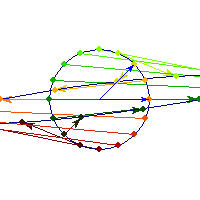Vectors are flipped across one axis only, so sometimes the angle is more than Pi/2 and sometimes it's less. A quadratic form corresponding to such matrix will have a saddle point (you can see this because x'Ax will be positive along some directions, and negative along others)

Positive definite but not symmetric

Rotation by less than Pi/2 and positive skew are positive definite operations, even though they are not symmetric or diagonalizable.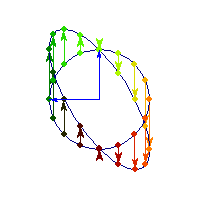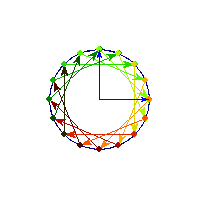You can see in the first case there's only one vector (0,1) that does't change direction after action by A, so there's only 1 eigenvector, hence the matrix is not diagonalizable. In the second case, every vector changes after action by A so there are no (real) eigenvectors, and matrix is again not diagonalizable.

## Saturday, April 29, 2006

### Naive Bayes vs. Logistic Regression

There's often confusion as to the nature of the differences between Logistic Regression and Naive Bayes Classifier. One way to look at it is that Logistic Regression and NBC consider the same hypothesis space, but use different loss functions, which leads to different models for some datasets.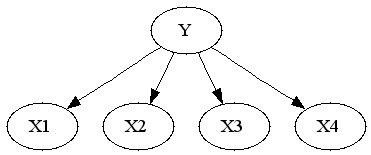To see that both logistic regression and naive bayes classifier consider the same hypothesis space we can rewrite Naive Bayes density as follows (restricting attention to binary domain):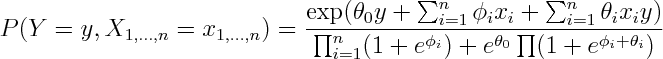where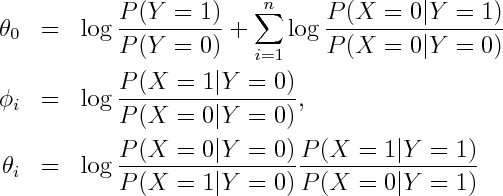Alternatively you can get exponential family parametrization from the observation that Naive Bayes model has no unshielded colliders, so undirected model obtained by dropping the arrows is equivalent on the set of positive densities.

From here you can rewrite it as product of conditional and marginal distributions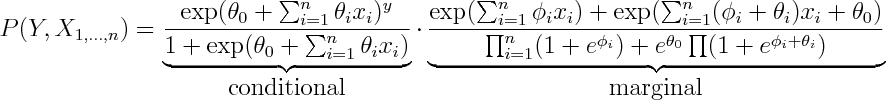You can see that the conditional term is equivalent to logistic regression, so every possible conditional density that can be modelled by logistic regression can be modelled by Naive Bayes by setting \phi's aribrarily.

So both logistic regression and Naive Bayes have the same hypothesis space, but optimize different objective functions. In particular logistic regression maximizes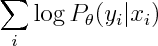whereas Naive Bayes maximizies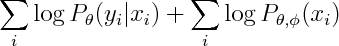You can see that the second term involves both phis and thetas, so conditional and marginal likelihoods are coupled. If empirical density is realizable by our model, then this coupling doesn't matter -- both conditional and marginal terms can achieve their respective maxima so Naive Bayes and Logistic Regression will produce the same estimate. However that not necessarily true when empirical density is unrealizable -- you may have to compromise between achieving high conditional likelihood and high marginal likelihood.

To get a generatively trained model that is identical to conditionally trained Naive Bayes, Minka suggests treating parameters in conditional and joint as separate sets.

So now we can introduce n new parameters so that conditional and marginal densities become decoupled.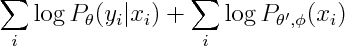It's interesting to see what is the new marginal density of our model. The marginal density marginalizes out Y. Since Y is the only separator in original graph, the resulting graph will be fully connected.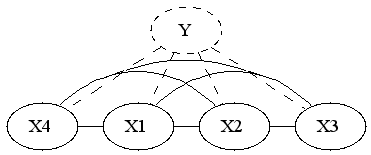So if we were to restrict attention to linear exponential families, we'd have to introduce 2^n features. I'm wondering if it's possible to infer that fact by looking at the form of the density. You'd have to show that the size of sufficient statistic is at least 2^n, or alternatively show that the smallest linear space that embeds the unnormalized log densities has dimension 2^n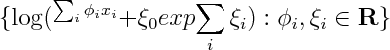If x_i are real or complex valued, one could show that the smallest linear space that embeds the set above has to be infinite dimensional, by noting that since log(1+exp(ax)) has singularities at i pi a, so one can always construct linearly independent elements by choosing a accordingly. I wonder, are there similar tricks that would work for discrete x?

(derivations)

## Monday, April 17, 2006

### Derivation of probability calculus

Here is an succinct derivation of probability calculus by Skillings (appeared in Appendix of his MaxEnt 2005 "Bayesics" article)

## Friday, March 31, 2006

### Graphical Models class notes

I needed to refresh some Graphical Models basics, and here are lecture notes I found useful
• Jeff Bilmes' Graphical Models course , gives detailed introduction on Lauritzen's results, proof of Hammersley-Clifford results (look in "old scribes" section for notes)
• Kevin Murphy's Graphical Models course , good description of I-maps
• Sam Roweis' Graphical Models course , good introduction on exponential families
• Stephen Wainwright's Graphical Models class, exponential families, variational derivation of inference
• Michael Jordan's Graphical Models course, his book seems to be the most popular for this type of class
• Lauritzen's Graphical Models and Inference class , also his Statistical Inference class , useful facts on exponential families, information on log-linear models, decomposability
• Donna Precup's class , some stuff on structure learning
Additionally, for information on directed/undirected graph equivalence classes, this paper by Meek is good, also this thesis gives some information for equivalence classes in ancestral/chain graphs

## Wednesday, March 22, 2006

### Challenge: Online Learning for Cell Phone MessagingIf you've used cell phones to send text messages you probably know about their auto-complete feature. For those that haven't -- each digit corresponds to 3 or 4 letters, you enter the digits consistent with your word, and it tries to guess which word you meant. For instance you enter "43556", and it will automatically guess "hello". But if you enter "785" to mean "SVM", it'll probably guess "run", and you'll have to back-up and re-enter the word. The challenge is for the phone to guess the right word.

Since new abbreviations spring up all the time, there's no dictionary that can cover all the words used in text-messages, so an ideal cell-phone will have an algorithm that can adapt to the user. The interesting question is how well can you do?

What makes this domain different from other sources of text is that it's a conversation, consisting of a series of short posts, containing colloquial grammar, bad spelling and abbreviations. I ran some simple algorithms on a similar dataset - 1.5M words of posts from a single person from an online chatroom.

The simplest algorithm is to return the most recent word encountered, consistent with given numbering. You can also return the most frequent word, or have a compound learner that returns one or the other. Cost of 1 is incurred every time an incorrect word is guessed. Here's what the curves look likeThe "best rate" curve represents the cost incurred if we only make errors on words that haven't been seen before. After 1.5M words, this "algorithm" makes mistakes about 20% of the time relative to the simple learners, which means there's a lot of room for improvement. And since this "algorithm" only sees 2-3 candidates for each word entered, there's no need to worry about efficient inference.

So here's a challenge -- can you approach the "best rate" curve any closer without using any other sources of text? (like dictionaries) If so, I'd like to hear from you! Here's the simplest script to generate an evaluation like above.

(BTW, I have several GB's of chatlogs, and I'll make more data available if there's demand)

## Friday, March 03, 2006

### Machine Learning videos

Learning from presentation or slides can be a much easier than from papers. I was reminded of that when I ran across Martin Wainwright's excellent class on Graphical Models. It has lecture notes *and* videos online.

Another large repository of machine learning related videos is the repository organized by the Pascal Network. You may run into a couple of bugs, but Sebastjan Mislej (sebastjan dot mislej dot ijs dot si) is very quick at fixing them when you email him.

Does anyone else have a favourite set of Machine Learning slides/notes/videos online?